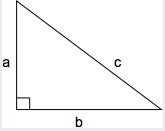According to the Pythagorean theorem, the hypotenuse squared is equal to the sum of the two perpendicular sides squared: $$c^2= a^2+ b^2 = 6^2 + 9^2 = 117$$
$$c= \sqrt{117}$$.